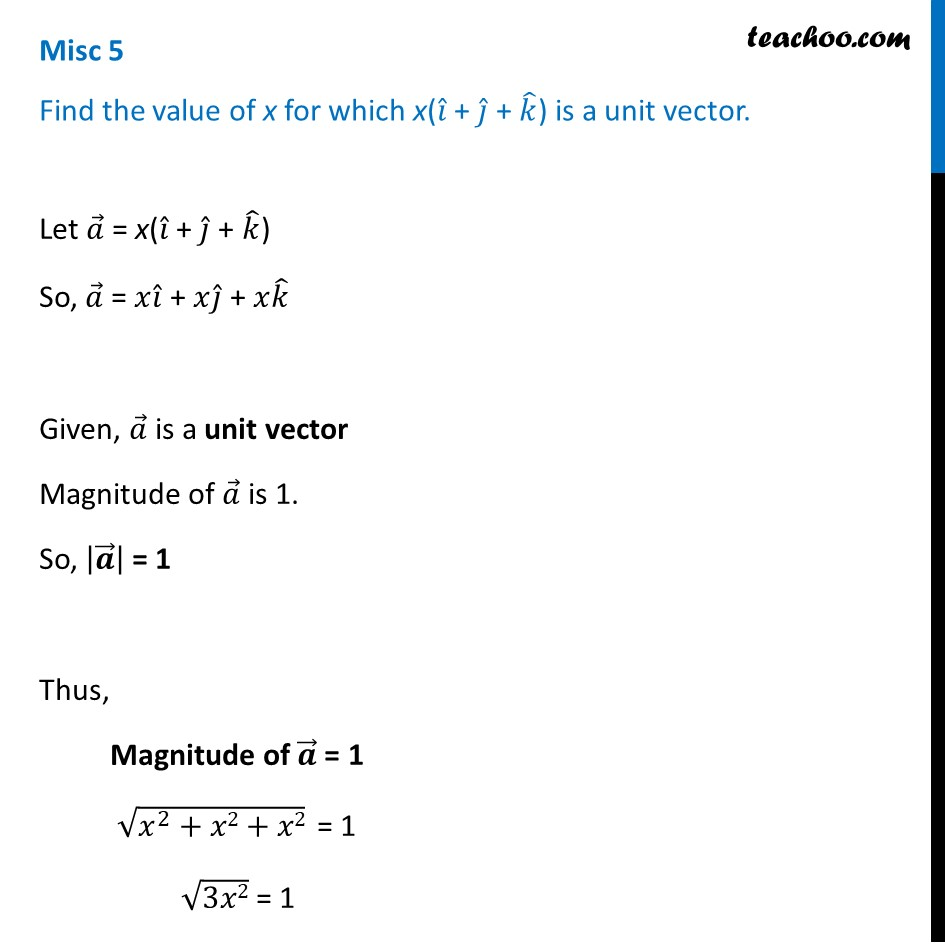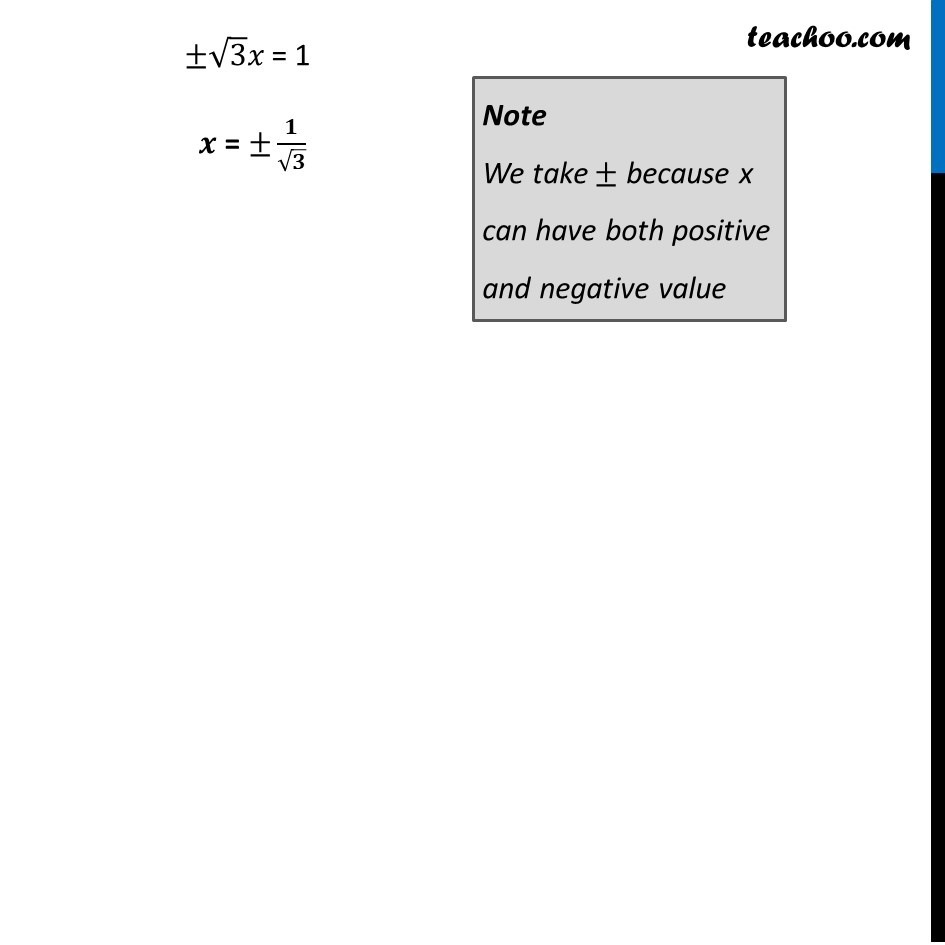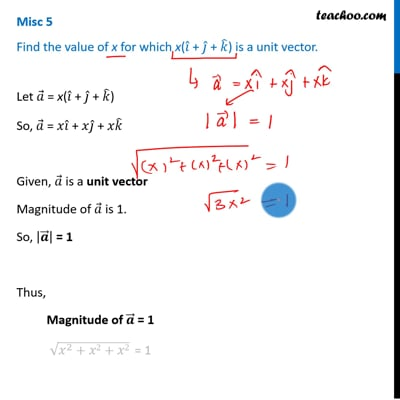Miscellaneous

Chapter 10 Class 12 Vector Algebra
Serial order wiseThis video is only available for Teachoo black users

Introducing your new favourite teacher - Teachoo Black, at only ₹83 per month

### Transcript

Misc 5 Find the value of x for which x(𝑖 ̂ + 𝑗 ̂ + 𝑘 ̂) is a unit vector.Let 𝑎 ⃗ = x(𝑖 ̂ + 𝑗 ̂ + 𝑘 ̂) So, 𝑎 ⃗ = 𝑥𝑖 ̂ + 𝑥𝑗 ̂ + 𝑥𝑘 ̂ Given, 𝑎 ⃗ is a unit vector Magnitude of 𝑎 ⃗ is 1. So, |𝒂 ⃗ | = 1 Thus, Magnitude of 𝒂 ⃗ = 1 √(𝑥^2+𝑥2+𝑥2) = 1 √3𝑥2 = 1 ±√3 𝑥 = 1 𝒙 = ±𝟏/√𝟑 Note We take ± because x can have both positive and negative value